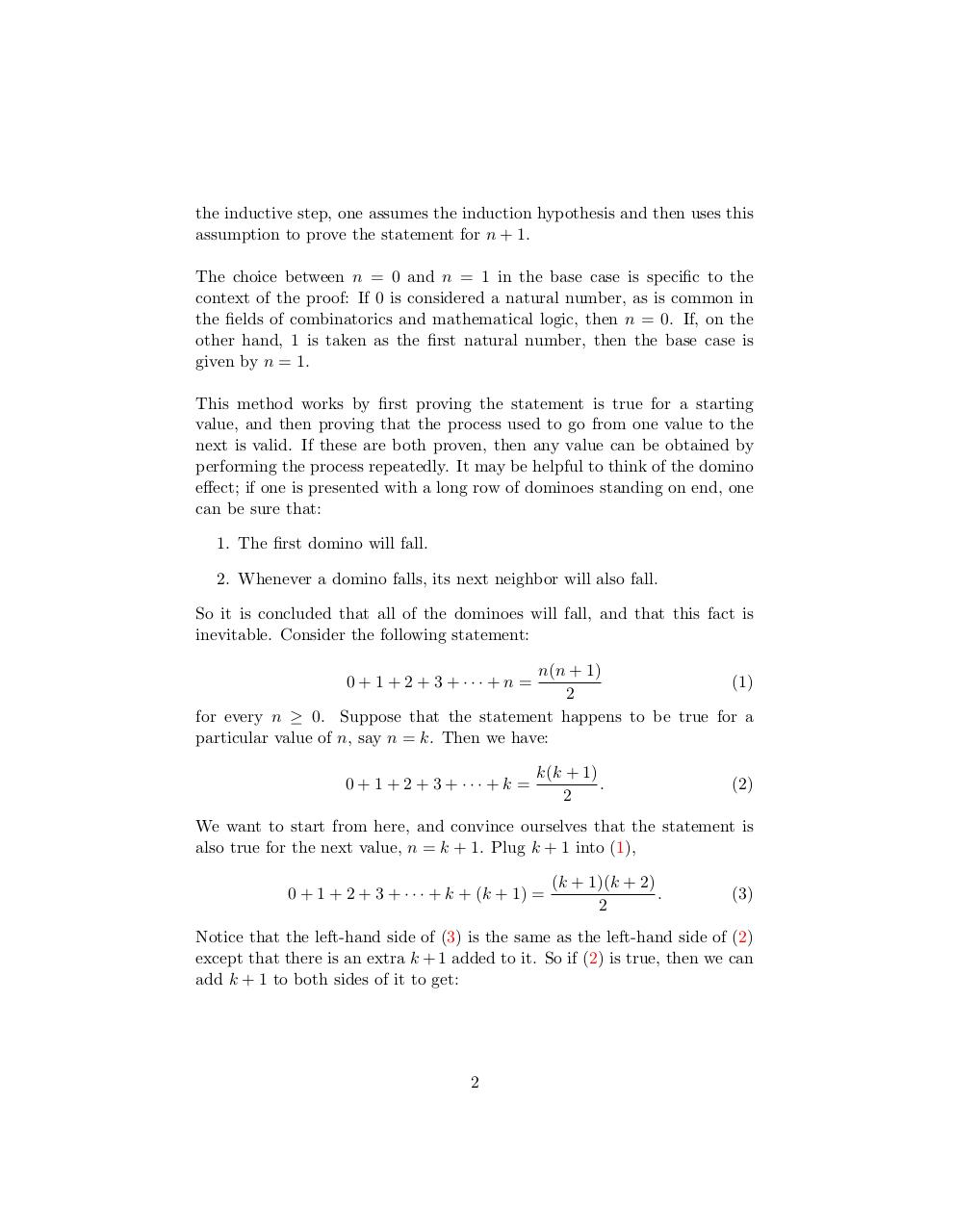# induction.pdfPage 1 2 3 4 5 6 7 8

#### Text preview

the inductive step, one assumes the induction hypothesis and then uses this
assumption to prove the statement for n + 1.
The choice between n = 0 and n = 1 in the base case is specific to the
context of the proof: If 0 is considered a natural number, as is common in
the fields of combinatorics and mathematical logic, then n = 0. If, on the
other hand, 1 is taken as the first natural number, then the base case is
given by n = 1.
This method works by first proving the statement is true for a starting
value, and then proving that the process used to go from one value to the
next is valid. If these are both proven, then any value can be obtained by
performing the process repeatedly. It may be helpful to think of the domino
effect; if one is presented with a long row of dominoes standing on end, one
can be sure that:
1. The first domino will fall.
2. Whenever a domino falls, its next neighbor will also fall.
So it is concluded that all of the dominoes will fall, and that this fact is
inevitable. Consider the following statement:
n(n + 1)
(1)
2
for every n ≥ 0. Suppose that the statement happens to be true for a
particular value of n, say n = k. Then we have:
0 + 1 + 2 + 3 + ··· + n =

0 + 1 + 2 + 3 + ··· + k =

k(k + 1)
.
2

(2)

We want to start from here, and convince ourselves that the statement is
also true for the next value, n = k + 1. Plug k + 1 into (1),
0 + 1 + 2 + 3 + · · · + k + (k + 1) =

(k + 1)(k + 2)
.
2

(3)

Notice that the left-hand side of (3) is the same as the left-hand side of (2)
except that there is an extra k + 1 added to it. So if (2) is true, then we can
add k + 1 to both sides of it to get:

2